# Demystifying Meshes

Back in 2011, I was working on Augmented Reality with Matt, Silka and John at Dekko, the spiritual predecessor of 6D.ai. That’s when, as a young software engineer, I faced an embarrassing problem - 3D meshes were everywhere, but they seemed like an esoteric black box to me. Does that sound familiar?

After seven years in the field, I’ve learned a lot and wanted to share those learnings with junior engineers facing the same problems. By the end of this article, you should have an understanding of the fundamentals of what meshes are, how to assemble them, how to manipulate them, and hopefully some experiment ideas to try in the beta 6D.ai SDK.

Grab a piece of paper. You might have a printer nearby, or a legal pad you can tear a page from, or a business card laying around. Now fold that into a boat, or a crane, or a plane.

Look at your creation. It is made of a number of faces, most of them triangles formed by the folds and edges of the piece of paper. See how those edges and folds cross to form new points on the sheet. By making a 3D object from well-defined flat faces, you created a 3D mesh.

In the digital world, the mesh creation process is reversed: points are created first and positioned in 3D space (we call them vertex), put in a list one after the other (a vertex array) so they each get a number (index). Only then, triangles (faces, or polygons) are created, each from three vertex indices. The reason is that a lot of vertices are used for multiple faces, and repeating their 3D coordinates for every triangle would be very inefficient in terms of memory usage.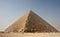Great Pyramid of Giza — by Nina from the Norwegian bokmål language Wikipedia

To illustrate this post, I will make a basic mesh based on the Great Pyramid of Giza, a very simple shape made of only four identical triangles. I'll be showing how it is represented in the .obj Waveform format (a basic but popular 3D model file format), in Unity (using C#), and in Apple's SceneKit (using Swift).

Let's talk about coordinate systems. There is no consensus on conventions, and different environments use a different handedness or pick a different axis to represent "up". SceneKit uses the right-handed OpenGL convention (X is right, Y is up, Z is towards the viewer), while Unity uses a left-handed variation (X is right, Y is up, Z is away from the viewer). For this example, I will use the Unity convention as we do in the 6D.ai SDK but I will place the origin at the center of the square base of the pyramid so that no transformation is needed for SceneKit thanks to the object's symmetry along the Z axis.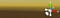Screenshot from the Unity editor showing the three axes of the coordinate system. Note that Z points away us.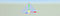Screenshot from Xcode's SceneKit editor showing the three axes of the coordinate system. Note that Z points towards us.

# Vertices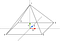Schematic representation of the five vertices, with 1 as the apex.

OBJ files describe the list of vertices with one vertex per line, each starting with the letter `v`. The first vertex will have index 1, the second one index 2, and so on.

`v 0.0 146.7 0.0v 115.17 0.0 115.17v 115.17 0.0 -115.17v -115.17 0.0 -115.17v -115.17 0.0 115.17`

Unity's C# packages vertices in a `Vector3[]` array.

`List<Vector3> vertices = new List<Vector3>();vertices.Add(new Vector3(0.0, 146.7, 0.0));vertices.Add(new Vector3(115.17, 0.0, 115.17));vertices.Add(new Vector3(115.17, 0.0, -115.17));vertices.Add(new Vector3(-115.17, 0.0, -115.17));vertices.Add(new Vector3(-115.17, 0.0, 115.17));Vector3[] vertexArray = vertices.ToArray();`

Swift/SceneKit packages vertices in a `[SCNVector3]` array that is then wrapped into a `SCNGeometrySource` object.

`var vertices : [SCNVector3] = []vertices.append(SCNVector3(0.0, 146.7, 0.0))vertices.append(SCNVector3(115.17, 0.0, 115.17))vertices.append(SCNVector3(115.17, 0.0, -115.17)) vertices.append(SCNVector3(-115.17, 0.0, -115.17))vertices.append(SCNVector3(-115.17, 0.0, 115.17))let vertexSource = SCNGeometrySource(vertices: vertices)`

# Faces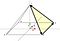Schematic representation of the pyramid highlighting the counter-clockwise first triangle (1, 3, 2).

OBJ files describe the list of faces with one face per line, each starting with the letter `f`.

`f 1 3 2f 1 4 3f 1 5 4f 1 2 5`

Unity’s C# packages triangles in a flat `int[]` array. Unlike OBJ files, vertex indices start at 0.

`List<int> triangles = new List<int>();triangles.AddRange(new[]{0, 2, 1});triangles.AddRange(new[]{0, 3, 2});triangles.AddRange(new[]{0, 4, 3});triangles.AddRange(new[]{0, 1, 4});int[] triangleArray = triangles.ToArray();`

Swift/SceneKit packages triangles in a `[Int32]` array that is then wrapped into a `SCNGeometryElement` object. Other more efficient representations exist, see SCNGeometryPrimitiveType. Like Unity/C#, vertex indices start at 0.

`var indices : [Int32] = []indices.append(contentsOf: [0, 2, 1])indices.append(contentsOf: [0, 3, 2])indices.append(contentsOf: [0, 4, 3])indices.append(contentsOf: [0, 1, 4])let element = SCNGeometryElement(indices: indices, primitiveType: .triangles)`

# Mesh Objects

Unity's C# packages vertices and triangles into a `Mesh` object. That object can then be added to a scene through the `MeshFilter`, `MeshRenderer` and `MeshCollider` components of a `GameObject` to achieve rendering, occlusion, and physics.

`// Create a Mesh object and assign vertices and triangles from aboveMesh mesh = new Mesh();mesh.vertices = vertexArray;mesh.triangles = triangleArray;// Create a GameObject in the sceneGameObject meshGameObject = new GameObject("Mesh GameObject");// Add a MeshFilter component, which is a mesh placeholder,// and attach the Mesh object to itMeshFilter meshFilter = meshGameObject.AddComponent<MeshFilter>();meshFilter.sharedMesh = mesh;// Add a MeshRenderer component, which renders the same GameObject's // MeshFilter's shared mesh with a Material of your choiceMeshRenderer meshRenderer = meshGameObject.AddComponent<MeshRenderer>();meshRenderer.material = new Material(Shader.Find("Standard"))// Add a MeshCollider component, which enables physics. Colliders// have their own shared mesh and do not use the MeshFilter's oneMeshCollider meshCollider = meshGameObject.AddComponent<MeshCollider>();meshCollider.sharedMesh = mesh;`

Swift/SceneKit packages vertices and triangles into a `SCNGeometry` object which is then attached to a `SCNScene`'s `SCNNode` (for rendering) or to that node's `SCNPhysicsBody` (for physics).

`// Create a SCNGeometry object and assign vertices and element// from abovelet geometry = SCNGeometry(sources: [vertexSource],                           elements: [element])// Attach a SCNMaterial of your choice to the geometrygeometry.firstMaterial = SCNMaterial()// Create a SCNNode in the SCNScenelet node = SCNNode()scene.rootNode.addChildNode(node)// Update the node's geometry for renderingnode.geometry = geometry// Update the node's physics body for collisionslet options = [SCNPhysicsShape.Option.keepAsCompound: true as Any,               .type: SCNPhysicsShape.ShapeType.concavePolyhedron]let shape = SCNPhysicsShape(geometry: geometry, options: options)let physicsBody = SCNPhysicsBody(type: .static, shape: shape)node.physicsBody = physicsBody`

As an exercise, try completing the scene with the two smaller pyramids:
apex 143.5m centered at (-320, -340), base 215.28m wide;
apex 65m centered at (-560, -740), base 102.2m by 104.6m wide.

In reality, mesh rendering and physics require additional data, such as normals or texture coordinates, in order to render light realistically, apply a texture to a face, simulate collisions with better fidelity.

Mesh rendering to is too complex a subject for a small blog post; however it is important to know how it works at a high level to understand why it needs this additional data.

Those parameters are used by programs running on the GPU called Shaders. Shaders have their own programming languages and are incredibly arcane to newcomers, so recently 3D platforms such as Unity and SceneKit have been providing their own one-size-fits-all shaders, making custom rendering more accessible through what-you-see-is-what-you-get options and parameters in materials.

The only thing you'll need to know about shaders, for now, is that their basic form has two steps: the Vertex Shader, which goes through every vertex of the mesh to calculate intermediate values (position, color, transparency, normal vector, texture coordinates, etc.); and the Fragment Shader, which goes through every pixel to calculate its final color, from the interpolation of the vertex shader's intermediate values.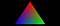The "Hello World" program for shaders is often a single triangle whose three edges have different colors, to illustrate how the pixels in the middle get interpolated colors.

Light in particular is dependent on Normals. A normal is the vector that's perpendicular to a plane, and it is used to calculate how directional light bounces off surfaces. The direction of normals can make a surface look smooth or reveal sharp edges, without any change of the mesh's geometry.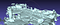In this screenshot of the 6D.ai office seen in MeshLab, perpendicular normals are used to give a uniform color to faces, highlighting edges with contrast.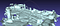In this screenshot of the same mesh with the same shader, averaged normals are used to create a shade gradient inside faces, camouflaging edges and giving the same polygons a smoother appearance.

# Normals

Let’s go back to our pyramid. In our case, we’ll want very sharp edges and a uniform brightness for each of the four face, depending on the position of the sun. This means that we'll calculate the normal vector for each face, and apply that normal for each of its three vertices.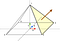Schematic representation of the pyramid highlighting the normal vector of the pyramid's first face with a brown arrow. Its value (0.703, 0.552, 0) will be applied to vertices 1, 2, and 3.

Normals are calculated using the cross product of two vectors, in the case of our first triangle (1, 3, 2) it is V(1→2) ⨯ V(1→3).

The order is important to get the normal's direction right; also the operands are inverted due to the left-handedness of the coordinate system. We will not detail the step-by-step calculation in this post, but it's simple enough to do with a piece of paper and a calculator as an exercise.

The normals we obtain for the four faces are, going clockwise around the pyramid and starting with the right face: (0.703, 0.552, 0.0), (0.0, 0.552, -0.703), (-0.703, 0.552, 0.0), (0.0, 0.552, 0.703). Note that the Y component is identical on all four of them, because all faces have the same slope.

OBJ files describe the list of normal vectors with one vector per line, each starting with the letters `vn`. The first vector will have index 1, the second one index 2, and so on. This list goes between the vertices and faces. Faces must then reference the relevant normal index after the vertex index, separated by `//`. In this format, vertices are independent from normals.

`vn 0.703 0.552 0.0vn 0.0 0.552 -0.703vn -0.703 0.552 0.0vn 0.0 0.552 0.703f 1//1 3//1 2//1f 1//2 4//2 3//2f 1//3 5//3 4//3f 1//4 2//4 5//4`

Unity’s C# packages normal vectors in a `Vector3[]` array, but there's a catch: you cannot use the same vertex with a different normal, so you need to duplicate it. In our case, that means we need to duplicate the apex vertex 4 times, for the normals of each face, and each corner twice. In this example, we do not get to reuse any vertex.

`List<Vector3> vertices = new List<Vector3>();// Right trianglevertices.Add(new Vector3(0.0, 146.7, 0.0));vertices.Add(new Vector3(115.17, 0.0, -115.17));vertices.Add(new Vector3(115.17, 0.0, 115.17));// Front trianglevertices.Add(new Vector3(0.0, 146.7, 0.0));vertices.Add(new Vector3(-115.17, 0.0, -115.17));vertices.Add(new Vector3(115.17, 0.0, -115.17));// Left trianglevertices.Add(new Vector3(0.0, 146.7, 0.0));vertices.Add(new Vector3(-115.17, 0.0, 115.17));vertices.Add(new Vector3(-115.17, 0.0, -115.17));// Back trianglevertices.Add(new Vector3(0.0, 146.7, 0.0));vertices.Add(new Vector3(115.17, 0.0, 115.17));vertices.Add(new Vector3(-115.17, 0.0, 115.17));Vector3[] vertexArray = vertices.ToArray();List<Vector3> normals = new List<Vector3>();// Right trianglenormals.Add(new Vector3(0.703, 0.552, 0.0));normals.Add(new Vector3(0.703, 0.552, 0.0));normals.Add(new Vector3(0.703, 0.552, 0.0));// Front trianglenormals.Add(new Vector3(0.0, 0.552, -0.703));normals.Add(new Vector3(0.0, 0.552, -0.703));normals.Add(new Vector3(0.0, 0.552, -0.703));// Left trianglenormals.Add(new Vector3(-0.703, 0.552, 0.0));normals.Add(new Vector3(-0.703, 0.552, 0.0));normals.Add(new Vector3(-0.703, 0.552, 0.0));// Back trianglenormals.Add(new Vector3(0.0, 0.552, 0.703));normals.Add(new Vector3(0.0, 0.552, 0.703));normals.Add(new Vector3(0.0, 0.552, 0.703));Vector3[] normalArray = normals.ToArray();List<int> triangles = new List<int>();triangles.AddRange(new[]{0, 1, 2});triangles.AddRange(new[]{3, 4, 5});triangles.AddRange(new[]{6, 7, 8});triangles.AddRange(new[]{9, 10, 11});int[] triangleArray = triangles.ToArray();// Create a Mesh object and assign vertices, normals, and triangles// from aboveMesh mesh = new Mesh();mesh.vertices = vertexArray;mesh.normals = normalArray;mesh.triangles = triangleArray;`

Swift/SceneKit packages normal vectors in a `[SCNVector3]` array that is then wrapped into a `SCNGeometrySource` object. Similarly to Unity, you need to duplicate vertices to use them with different normals.

`var vertices : [SCNVector3] = []// Right trianglevertices.append(SCNVector3(0.0, 146.7, 0.0))vertices.append(SCNVector3(115.17, 0.0, -115.17))vertices.append(SCNVector3(115.17, 0.0, 115.17))// Front trianglevertices.append(SCNVector3(0.0, 146.7, 0.0))vertices.append(SCNVector3(-115.17, 0.0, -115.17))vertices.append(SCNVector3(115.17, 0.0, -115.17))// Left trianglevertices.append(SCNVector3(0.0, 146.7, 0.0))vertices.append(SCNVector3(-115.17, 0.0, 115.17))vertices.append(SCNVector3(-115.17, 0.0, -115.17))// Back trianglevertices.append(SCNVector3(0.0, 146.7, 0.0))vertices.append(SCNVector3(115.17, 0.0, 115.17))vertices.append(SCNVector3(-115.17, 0.0, 115.17))let vertexSource = SCNGeometrySource(vertices: vertices)var normals : [SCNVector3] = []// Right trianglenormals.append(SCNVector3(0.703, 0.552, 0.0))normals.append(SCNVector3(0.703, 0.552, 0.0))normals.append(SCNVector3(0.703, 0.552, 0.0))// Front trianglenormals.append(SCNVector3(0.0, 0.552, -0.703))normals.append(SCNVector3(0.0, 0.552, -0.703))normals.append(SCNVector3(0.0, 0.552, -0.703))// Left trianglenormals.append(SCNVector3(-0.703, 0.552, 0.0))normals.append(SCNVector3(-0.703, 0.552, 0.0))normals.append(SCNVector3(-0.703, 0.552, 0.0))// Back trianglenormals.append(SCNVector3(0.0, 0.552, 0.703))normals.append(SCNVector3(0.0, 0.552, 0.703))normals.append(SCNVector3(0.0, 0.552, 0.703))let normalSource = SCNGeometrySource(normals: normals)var indices : [Int32] = []indices.append(contentsOf: [0, 1, 2])indices.append(contentsOf: [3, 4, 5])indices.append(contentsOf: [6, 7, 8])indices.append(contentsOf: [9, 10, 11])let element = SCNGeometryElement(indices: indices, primitiveType: .triangles)// Create a SCNGeometry object and assign vertices, normals,// and element from abovelet geometry = SCNGeometry(sources: [vertexSource, normalSource],                           elements: [element])`

Another common value encoded per vertex is Texture Coordinates or UVs (not to be confused with the UV channels of the YUV color space). We won't cover those in details, but the high level idea is that textures are sampled with logical floating point coordinates between 0.0 and 1.0 on both axes. This allows artists to paint the different parts of a complex 3D model with a single 2D image.

If you still have your origami creation handy, unfold it: the corners between edges and folds are your UV coordinates.

Now that we've covered in details what a mesh is made of, let's tie it all to the 6D.ai beta SDK. If you were to scan the Great Pyramid with our SDK, you wouldn't obtain the four identical triangles from the example. The actual mesh would be millions, if not billions, of small triangles, closely wrapping the imperfect surface of the pyramid.

Currently, the 6D.ai beta SDK API calls provide mesh information for up to 10 meters (32 feet) around the user. In order to facilitate mesh manipulation, we organize this "triangle soup" into mesh blocks. We slice the world into cubes of 22.4cm by 22.4cm by 22.4cm (8.82in), and every cube that has at least one triangle of mesh in it is a block.

# Mesh Blocks

• (X, Y, Z) block coordinates
(0, 0, 0) is the coordinates of the block containing vertices and triangle between 0.0 and 0.224 on every axis; (-1, -1, -1) is the block containing vertices and triangles between -0.224 and 0.0 on every axis.
• a vertex count
• a face count
• a version number
Updating mesh objects is expensive, so the version number only increases when its vertices or faces update.

The block size was chosen as a compromise between noise and precision. The underlying depth detection models the world in small cubes known as voxels, and estimates for each a probability to be void or solid. The mesh is made from the surface separating solid and empty voxels, block per block, each block containing 512 individual voxels, corresponding to the amount of parallel processing used by our algorithms.

In the sample apps of the 6D.ai beta SDK, the mesh is used for rendering and occlusion, but also for physics and collisions. Updating collision meshes is an expensive operation, which grows linearly with the polygon count. We limit the frequency and cost of collision meshes by only updating them when we know they changed (thanks to block versions), and by splitting them into smaller sub-meshes.

Unfortunately, each sub-mesh comes with overhead, so we limit their number on the application side by grouping blocks into chunks, which are larger groups of about 1m (4ft) a side.

# Mesh API

`SixDegreesSDK_GetMeshBlockInfo()` returns four integer values (the last three are passed as `int*` parameters):

• a version number
Similar to the block version, but applied to the entire mesh. If this number matches the previous one, you are guaranteed the mesh hasn't changed. If this value is negative, no mesh data is available, and if this value is zero, the mesh has been reset.
• block buffer size
The size of the `int[]` array you need to allocate on the application side, to be populated by `SixDegreesSDK_GetMeshBlocks()`. Because the block count can be big, always allocate on the heap, not on the stack (think `malloc()` not `int blocks[bufferSize]`).
• vertex buffer size
Similarly, the size of the `float[]` array you need to allocate.
• face buffer size
The size of the `int[]` array you need to allocate.

Once your three buffers are ready, you can call `SixDegreesSDK_GetMeshBlocks()` and pass the three pointers to the buffers along with their respective sizes. This API calls only performs `memcpy()` from internal buffers and returns fast. Its return value is the number of blocks that are fully populated, if successful, and -1 otherwise if one of the buffers is too small.

# Parsing Mesh Buffers

Each six floats in the vertex buffer describe one vertex: X coordinate, Y coordinate, Z coordinate, X normal, Y normal, Z normal. If the first block has 3 vertices in it, then the first 3×6=18 floats in the vertex buffer are part of that block. Unfortunately, there is no UV coordinates and no texture at the moment.

Each three integers in the face buffer describe one face: first vertex index, second vertex index, third vertex index. Those indices pertain to the whole vertex buffer; if you cut the mesh into submeshes you'll have to offset them.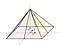Schematic representation of the pyramid, with each face tinted and split in the middle.

Let's say you are meshing a 1:1000 model of the Great Pyramid, and that the 6D SDK meshes its faces perfectly. The pyramid, being centered on the origin, spans four blocks, clockwise and starting from the corner to the right and away: (0, 0, 0), (0, 0, -1), (-1, 0, -1), (-1, 0, 0).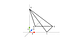Schematic representation of the first block in the mesh, made of 4 vertices and 2 faces. Vertex indices start at 0 in the 6D.ai SDK.

Since every triangle has to be fully contained inside a block, each side of the pyramid is split between two blocks. Each of the four blocks then gets four vertices and two faces. The 6D.ai mesh surface is designed to appear smooth, so normals are calculated per vertex based on the neighboring faces, differing from our theoretical full-scale Great Pyramid.

Overall, the mesh will have four blocks, 16 vertices and 8 faces. A call to `SixDegreesSDK_GetMeshBlockInfo()` would return: version = 1, blockBufferSize = 24, vertexBufferSize = 96, faceBufferSize = 24.

A call to `SixDegreesSDK_GetMeshBlockInfo()` would populate the buffers with the following values: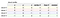The memory layout of the block buffer is represented on six columns here to show one block per line. Cells are colored per block to help find corresponding vertices and faces in the next two buffers.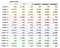Vertices at the boundaries of blocks are found multiple time, for instance the pyramid's apex is found as vertex index 0, 4, 8 and 12.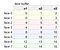In practice, blocks contain more than a hundred faces each, on average.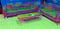Mesh at the front desk of the 6D.ai office, color coded by normals.

# Mesh Manipulation

This topic is incredibly important for the future of AR experiences, and will be the subject of several future articles. For today, I'll focus on a simple but powerful technique enabled by the 6D.ai mesh.

What if we wanted to cover the floor with colorful flowers? All we need is to find the 3D coordinates where we want to place them. This is made easy with mesh blocks.

The first step is to wait for a minimum number of blocks to appear, let's say 50, so there is enough data to work with. The number of blocks can be easily calculated from the block buffer size returned by `SixDegreesSDK_GetMeshBlockInfo()`: `int blockCount = blockBufferSize / 6;`

The second thing is to build a histogram of the blocks' Y coordinate. This assumes that as the floor gets meshed, the dominant Y coordinate will be the floor's. The assumption hinges on user motion, of course, so you may want to show on-screen cues to encourage the scanning of the floor. From there, you may use heuristics starting from the phone's current position (with `SixDegreesSDK_GetPose()`) and assume the floor will be somewhere roughly between 0 and 2 meters below the camera.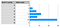In this example, there is a strong evidence of floor blocks being at the Y coordinate -5, further reinforced by the absence of lower blocks.

Then, in order to find the exact elevation of the floor, an approach is to sample a few vertices from blocks whose Y coordinate matches the dominant one found in the histogram. Floor vertices would face up, meaning their normal would be close to (0.0, 1.0, 0.0). You could collect a few Y vertex coordinates from each floor block, and pick a median value. Unless the floor is slanted, that one would be really close to the exact Y elevation of the floor.

Last, in order to perform the flower placement, you could iterate through blocks once more and randomly pepper instances of your 3D assets at the Y elevation of the floor. This is the basic idea of procedural placement based on topology.

Possibilities are endless. How would you detect a table? Or a wall? How would you support an uneven floor, like in a garden? How would you plan the path of a virtual pet playing fetch?

Future articles will explore those problems in detail. What would you like to do with your mesh? Please suggest ideas in the comments below.

# Conclusion

Written by

Written by

## Anthony Maës

#### Software Engineer at Niantic Labs / ex 6D.ai / antho.nyma.es